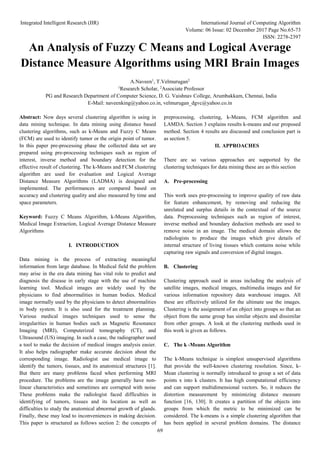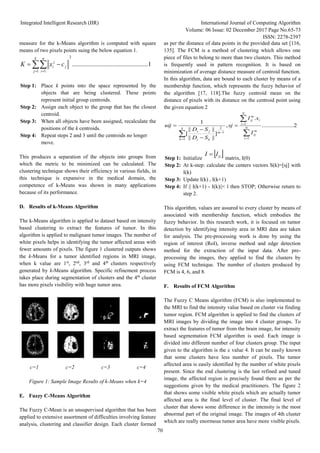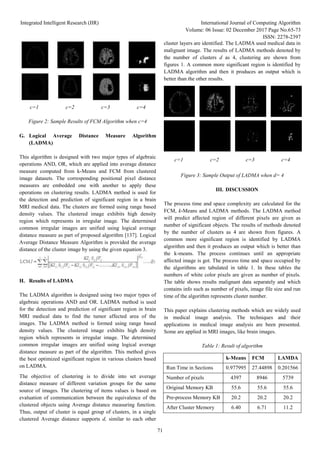Successfully reported this slideshow.

# An Analysis of Fuzzy C Means and Logical Average Distance Measure Algorithms using MRI Brain Images×

# An Analysis of Fuzzy C Means and Logical Average Distance Measure Algorithms using MRI Brain Images

Abstract: Now days several clustering algorithm is using in data mining technique. In data mining using distance based clustering algorithms, such as k-Means and Fuzzy C Means (FCM) are used to identify tumor or the origin point of tumor. In this paper pre-processing phase the collected data set are prepared using pre-processing techniques such as region of interest, inverse method and boundary detection for the effective result of clustering. The k-Means and FCMclustering algorithm are used for evaluation and Logical Average Distance Measure Algorithms (LADMA) is designed and implemented. The performances are compared based on accuracy and clustering quality and also measured by time and space parameters.

Abstract: Now days several clustering algorithm is using in data mining technique. In data mining using distance based clustering algorithms, such as k-Means and Fuzzy C Means (FCM) are used to identify tumor or the origin point of tumor. In this paper pre-processing phase the collected data set are prepared using pre-processing techniques such as region of interest, inverse method and boundary detection for the effective result of clustering. The k-Means and FCMclustering algorithm are used for evaluation and Logical Average Distance Measure Algorithms (LADMA) is designed and implemented. The performances are compared based on accuracy and clustering quality and also measured by time and space parameters.